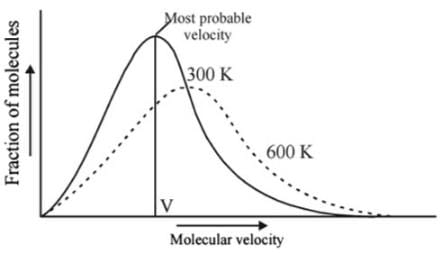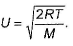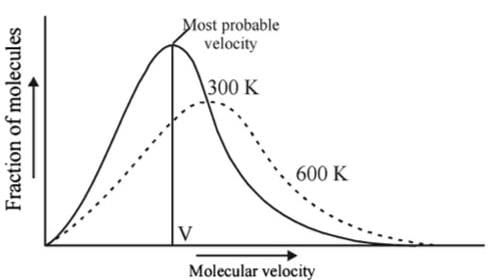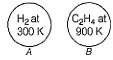NEET  >  Test: Kinetic Molecular Theory

# Test: Kinetic Molecular Theory

Test Description

## 19 Questions MCQ Test Topic-wise MCQ Tests for NEET | Test: Kinetic Molecular Theory

Test: Kinetic Molecular Theory for NEET 2022 is part of Topic-wise MCQ Tests for NEET preparation. The Test: Kinetic Molecular Theory questions and answers have been prepared according to the NEET exam syllabus.The Test: Kinetic Molecular Theory MCQs are made for NEET 2022 Exam. Find important definitions, questions, notes, meanings, examples, exercises, MCQs and online tests for Test: Kinetic Molecular Theory below.
Solutions of Test: Kinetic Molecular Theory questions in English are available as part of our Topic-wise MCQ Tests for NEET for NEET & Test: Kinetic Molecular Theory solutions in Hindi for Topic-wise MCQ Tests for NEET course. Download more important topics, notes, lectures and mock test series for NEET Exam by signing up for free. Attempt Test: Kinetic Molecular Theory | 19 questions in 19 minutes | Mock test for NEET preparation | Free important questions MCQ to study Topic-wise MCQ Tests for NEET for NEET Exam | Download free PDF with solutions
 1 Crore+ students have signed up on EduRev. Have you?
Test: Kinetic Molecular Theory - Question 1

### Direction (Q. Nos. 1-11) This section contains 11 multiple choice questions. Each question has four choices (a), (b), (c) and (d), out of which ONLY ONE option is correct. Q. Helium atom is two times heavier than a hydrogen molecule (H2). At 298 K, the average kinetic energy of helium atom is

Detailed Solution for Test: Kinetic Molecular Theory - Question 1

Kinetic energy of gas molecule depends on temperature and does not depend upon the nature of gas.

K.E =(3/2)kT.

Thus hydrogen and helium have same K.E at same temperature.

Test: Kinetic Molecular Theory - Question 2

### Mass of gas is 300 gm and its specific heat at constant volume is 750J/kg K. if gas is heated through 75°C at constant pressure of 105 N/m2, it expands by volume 0.08 × 106 cm3. find CP/CV.

Detailed Solution for Test: Kinetic Molecular Theory - Question 2

Data given;
ΔT = 75 oC = 75 K
ΔV = 0.08 * 106 cm3 = 0.08 m3
Cv  = 750 J kg-1 K-1
m = 300 g = 0.300 kg
p  = 105 N m-2 = 100000 N m-2
The first law of thermodynamics;
ΔU = Q – W
Q = ΔU + W    ---- (1)
mCpΔT = mCvΔT + pΔV   ----- (2)
Dividing the above expression (2) with Cv , we get;
Cp / Cv = Cv / Cv + pΔV / mΔTCv
Cp / Cv = 1 + pΔV / mΔTCv
Cp / Cv = 1 + ([100000 N m-2 . 0.08 m3] /                 [0.300 kg . 75 K .750 J kg-1K-1])
Cp / Cv = 1 + 0.474
Cp / Cv = 1.474 ; After rounding it off we get,
= 1.5 (appx)

Test: Kinetic Molecular Theory - Question 3

### At what temperature will the collision frequency in N2 be equal to that in He at 25°C if both gases are at a pressure of 1.00 bar collision diameter = 0.373 nm for N2 = 0.218 nm for He (Assume equal number of molecules per unit volume.)

Detailed Solution for Test: Kinetic Molecular Theory - Question 3

Collision frequency=RMSvelocity/mean free path= √3RT/M/kT/ √2σ2p

R=gas constant=8.314J/K/mol

T=temperature

M=molecular mass

K=boltzmann constant

P=pressure, σ=collision constant

Collision frequency of nitrogen/ Collision frequency of nitrogen=[√3RT/M]/[kT/√2 σ2p] nitrogen/[√3RT/M]/[kT√2 σ2p]helium

As the collision frequency is same

=>[√3RT/M]/[kT/√2 σ2p] nitrogen=[√3RT/M]/[kT√2 σ2p]helium

=>[√T/M/T/ σ2p] nitrogen=[√T/M/T/ σ2p] helium

=> [σ2/M√T] nitrogen=[[σ2/M√T]helium

=0.373/18x√T=0.218/4x√298

=>T=17.80K

Test: Kinetic Molecular Theory - Question 4

According to the kinetic theory of gases, in an ideal gas, between two successive collisions a gas molecule travels

[IIT JEE 2003]

Detailed Solution for Test: Kinetic Molecular Theory - Question 4

According to kinetic theory of gases, a gas molecule travels in a straight line path.

Test: Kinetic Molecular Theory - Question 5

As the tem perature is raised from 20°C to 40°C. The average kinetic energy of the neon atoms changes by a factor of which of the following?

[IIT JEE 2004]

Detailed Solution for Test: Kinetic Molecular Theory - Question 5

Average kinetic energy ∝ Temperature in Kelvin

(KE40) / (KE20) = (3/2 nR * 313)/(3/2 nR*293)

KE40 / KE20 = T2/T1 = 40 + 273 / 20 + 273  = 313/293

Test: Kinetic Molecular Theory - Question 6

Which one of the following statements is not true about the effect of an increase in temperature on the distribution of molecular speeds in a gas?

[IIT JEE 2005]

Detailed Solution for Test: Kinetic Molecular Theory - Question 6

Distribution of molecular velocities at two different temperature is shown below:-At higher temperature, moree molecules have higher velocities and less molecules have lower velocities.
From the above figure, it is clear that with the increase in temperature, most probable velocity increases but the fraction of such molecules decreases,
So option c is correct.

Test: Kinetic Molecular Theory - Question 7

Bond energy of H2 gas is 104 kcal mol-1. The temperature at which average kinetic energy of gaseous H2 molecules is equal to energy required to dissociate the molecules into atoms, is

Detailed Solution for Test: Kinetic Molecular Theory - Question 7

Bond energy is the energy to break bonds and convert the sample into atoms.
We know that,
K.E of a mol of gas molecule = 3/2 RT
A/Q 3/2 RT = 104 kcal mol-1
3/2×8.314×T = 104×103 x 4.18 J
T = 34893 K

Test: Kinetic Molecular Theory - Question 8

Compressibility factor for CO2 at 400 K and 71.0 bar is 0.8697. Molar volume of CO2 under these conditions is

Detailed Solution for Test: Kinetic Molecular Theory - Question 8

We know that
Z = pVobserved /RT
0.8697   =   71×0.987×Vobserved / 0.0821×400
Vobserved = 0.8697×0.0821×400/71×0.987
= 0.406 L or 0.41 L

Test: Kinetic Molecular Theory - Question 9

At what temperature will the hydrogen molecules have the same kinetic energy per mole as nitrogen molecules at 280 K?

Detailed Solution for Test: Kinetic Molecular Theory - Question 9

KE = 3/2 nRT
for 1 mole KE=3/2 RT

It is independent of the molecular mass and constant for a particular temperature.

Therefore T will be same 280K

Test: Kinetic Molecular Theory - Question 10

Temperature at which average translation energy of a molecule is equal to the kinetic energy of the electron in first Bohr is orbit is

Detailed Solution for Test: Kinetic Molecular Theory - Question 10

Av. Translational Energy of a molecule= 3/2 KT -------(1)
Kinetic Energy of the electron in first Bohr's orbit= 13.6eV = 13.6×1.6×10-19J--------(2)
from eqn. 1&2
3/2KT=13.6×1.6×10-19
T = 1.05×105 K

Test: Kinetic Molecular Theory - Question 11

Pressure (p) of an ideal gas is related to kinetic energy (E) per unit volume by equation

Detailed Solution for Test: Kinetic Molecular Theory - Question 11

We know that
K.E = 3/2RT = 3/2pV
Dividing both sides by V
K.E/V = 3/2p
E = 3/2p     (here E is K.E per unit volume)
Or p = 2/3E

Test: Kinetic Molecular Theory - Question 12

Direction (Q. Nos. 12 and 13) This sectionis based on statement I and Statement II. Select the correct answer from the code given below.

Q.

Statement I : The pressure exerted by a gas is the result of collisions of the molecules with the walls of the container.

Statement II : Due to collision, momentum is changed and rate of change of momentum is equal to force.

Detailed Solution for Test: Kinetic Molecular Theory - Question 12

Both statements are correct and statement 2 is the correct explanation of statement 1. The collisions of the molecules among themselves and with the walls of the container are perfectly elastic. Therefore, momentum and kinetic energy of the molecules are conserved during collisions.

Test: Kinetic Molecular Theory - Question 13

Statement I : As velocity increases, distribution of molecules increases and is maximum at most probable velocity, after which distribution of molecules decreases.

Statement II : Most probable velocity is given byDetailed Solution for Test: Kinetic Molecular Theory - Question 13

Both statements are correct but statement 2 is not the correct explanation of 1.Velocity distribution follows this graph and not the most probable formula.

*Multiple options can be correct
Test: Kinetic Molecular Theory - Question 14

Direction (Q. Nos. 14-15) This section contains 4 multiple choice questions. Each question has four choices (a), (b), (c) and (d), out of which ONE or  MORE THANT ONE  is correct.

Q. If a gas is expanded at constant temperature

Detailed Solution for Test: Kinetic Molecular Theory - Question 14

At constant temperature, P is inversely proportional to V. So for expansion, V increases and so P decreases. KE is 3/2 kT (for one atoom) or 3/2RT(for 1 mole). And with constant temperature, KE also remains constant.

*Multiple options can be correct
Test: Kinetic Molecular Theory - Question 15

According to kinetic theory of gases

[ITT JEE 2011]

Detailed Solution for Test: Kinetic Molecular Theory - Question 15

According to me, all statements are correct.
As per the postulates of the kinetic theory of gases, the molecules of gas collide with each other and with the walls of the container. These collisions are perfectly elastic in nature.
The gas molecule with mass m and speed u collides with the walls of the container to transfer the momentum Δp=−2mu. Thus heavier molecules transfer more momentum to the walls of the container. But this is not the postulate of the kinetic theory of gases.
As per Maxwell -Boltzmann distribution of molecular speed, very few molecules move with very high or very low speed. Most of the molecules move with average speed. Hence, only a small number of molecules have very high
velocity.
According to the kinetic theory of gases, a gas molecule moves in straight line with constant velocity. When it collides with another molecule, it changes direction. Between collisions, the molecules move in straight lines with constant velocities.

Test: Kinetic Molecular Theory - Question 16

Direction (Q. Nos. 16-19) This section contains a paragraph, wach describing theory, experiments, data etc. three Questions related to paragraph have been given.Each question have only one correct answer among the four given options (a),(b),(c),(d).

Passage I

Volume occupied by molecules is negligible compared to total volume of molecules. We have N2 gas molecules assuming them spherical of radius 200 pm.

Q.
Total volume of molecules contained in one mole of the gas is

Detailed Solution for Test: Kinetic Molecular Theory - Question 16

Volume of single molecule = 4/3π(r)3
= 4/3×π×(200×10-12)3 m3
V = 3.349×10-29 m3
Volume of 1 mole (NA molecules) =  3.349×10-29 m3×6.022×1023
= 2.016×10-5 m3
= 2.016×10-5×106 cm3 or 20.16 cm3

Test: Kinetic Molecular Theory - Question 17

Passage I

Volume occupied by molecules is negligible compared to total volume of molecules. We have N2 gas molecules assuming them spherical of radius 200 pm.

Q. Percentage of empty space available at STP is

Detailed Solution for Test: Kinetic Molecular Theory - Question 17

volume of one molecule of Nitrogen = 4/3πr3
= 4/3 x 3.14 x ( 2 x 10-10)3
= 3.351 x 10-29 m3
volume of one mole of N2 molecules
= 3.351 x 10-29 x 6.023 x 1023 m3
= 2.018 x 10-5 m3
volume of one mole of N2 gas = 22.4 Litre at NTP = 22.4 x 10-3 m3
space occupied by the molecules = 2.018x10-5/22.4x103x100
= 0.09 %
Empty space = 100 - 0.09 = 99.91 %

Test: Kinetic Molecular Theory - Question 18

Passage lI

Two flasks A and B have equal volumes as shown Both gases occupy 22.40 dm3 at STP. Also collision diameter of C2H4 molecule is four times than that of H2 molecule.Q. In which flask and how many times are molecules moving faster?

Detailed Solution for Test: Kinetic Molecular Theory - Question 18

(VAvg)a = √(8RT/πM)
On potting the value of condition of A and B, we get
VH2 = k(12.24)
VC2H4 = k(5.65)
Thus, molecules in H2 are moving 2.16 times faster.

Test: Kinetic Molecular Theory - Question 19

Passage lI

Two flasks A and B have equal volumes as shown Both gases occupy 22.40 dm3 at STP. Also collision diameter of C2H4 molecule is four times than that of H2 molecule.Q. In which flask and how many times is the mean free path of the molecules greater?

## Topic-wise MCQ Tests for NEET

1493 tests
 Use Code STAYHOME200 and get INR 200 additional OFF Use Coupon Code
Information about Test: Kinetic Molecular Theory Page
In this test you can find the Exam questions for Test: Kinetic Molecular Theory solved & explained in the simplest way possible. Besides giving Questions and answers for Test: Kinetic Molecular Theory, EduRev gives you an ample number of Online tests for practice

## Topic-wise MCQ Tests for NEET

1493 tests

### How to Prepare for NEET

Read our guide to prepare for NEET which is created by Toppers & the best Teachers